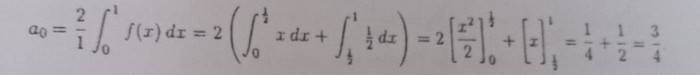# Half range sine series

• foo9008

## Homework Statement

f(x) = x , 0 <x<1/2
1/2 , 1/2 < x <1
in this question , I am not convinced that a_ 0 = 0 for half range sine series , because i found thatbut , thoerically , for half rang sine series , a_ 0 must be = 0 , ,am i right ? why the value of A- 0 that i got is not = 0 ? what's wrong with my working ?

## The Attempt at a Solution

Your calculation of ##a_0## is for the half range cosine series. It represents the even extension of your function. The half range sine series represents the odd extension of your function. They aren't the same thing.

Your calculation of ##a_0## is for the half range cosine series. It represents the even extension of your function. The half range sine series represents the odd extension of your function. They aren't the same thing.
sorry , can you explain further ? i did http://i.imgur.com/I5muGdJ.jpg
because i want to show that for half rang sine series , a_0 = 0 , however , i get the value of a_ 0 not equal to 0 ... what's wrong with my working ?

There is nothing wrong with your calculations. They are FS of different functions. If we call the even extension of your ##f(x)## by ##f_{even}(x)##, its equation on ##(-1,1)## is$$f_{even}(x) = \left \{ \begin{array}{r,l} \frac 1 2,&-1<x<-\frac 1 2\\ -x, & -\frac 1 2 < x < 0\\ x, & 0 < x < \frac 1 2 \\ \frac 1 2, &\frac 1 2 < x < 1 \end{array}\right .$$
Similarly, the odd extension ##f_{odd}(x)## is given by$$f_{odd}(x) = \left \{ \begin{array}{r,l} -\frac 1 2,&-1<x<-\frac 1 2\\ x, & -\frac 1 2 < x < \frac 1 2\\ \frac 1 2, &\frac 1 2 < x < 1 \end{array}\right .$$These are both defined on ##(-1,1)## and of period ##2##. If you do the full range FS of ##f_{even}## you will find out that ##b_n=0## and you get only ##a_n## nonzero terms, and ##a_0## is what you have calculated. That's what the half range cosine series is.

If you expand ##f_{odd}## as a full range FS you will find all ##a_n = 0## and only ##b_n##'s are non-zero.

The half range formulas are just shortcuts for these two series taking advantage of the even and oddness. They are two different functions. But note they both represent the same thing on ##(0,1)##.

•foo9008
There is nothing wrong with your calculations. They are FS of different functions. If we call the even extension of your ##f(x)## by ##f_{even}(x)##, its equation on ##(-1,1)## is$$f_{even}(x) = \left \{ \begin{array}{r,l} \frac 1 2,&-1<x<-\frac 1 2\\ -x, & -\frac 1 2 < x < 0\\ x, & 0 < x < \frac 1 2 \\ \frac 1 2, &\frac 1 2 < x < 1 \end{array}\right .$$
Similarly, the odd extension ##f_{odd}(x)## is given by$$f_{odd}(x) = \left \{ \begin{array}{r,l} -\frac 1 2,&-1<x<-\frac 1 2\\ x, & -\frac 1 2 < x < \frac 1 2\\ \frac 1 2, &\frac 1 2 < x < 1 \end{array}\right .$$These are both defined on ##(-1,1)## and of period ##2##. If you do the full range FS of ##f_{even}## you will find out that ##b_n=0## and you get only ##a_n## nonzero terms, and ##a_0## is what you have calculated. That's what the half range cosine series is.

If you expand ##f_{odd}## as a full range FS you will find all ##a_n = 0## and only ##b_n##'s are non-zero.

The half range formulas are just shortcuts for these two series taking advantage of the even and oddness. They are two different functions. But note they both represent the same thing on ##(0,1)##.
do you mean to get a_ 0 = 0 , we have to do the full range sine series ... so , we just need to follow the book stated that for half range sine series , a_ 0 = 0 ?
we wouldn't get a_ 0 = 0 if we do half range sine series only ?

do you mean to get a_ 0 = 0 , we have to do the full range sine series ... so , we just need to follow the book stated that for half range sine series , a_ 0 = 0 ?

You just need to understand that when you do a half range sine expansion of ##f(x)##, you are really doing the full range expansion of ##f_{odd}(x)##. But ##f_{odd}(x)## is an odd function, so its FS will have only nonzero ##b_n## and you don't have to calculate the ##a_n##. The advantage of using the half range formula for ##b_n## is that the integral only involves ##f(x)## so you don't have to figure out the full formula for ##f_{odd}(x)##.

•foo9008